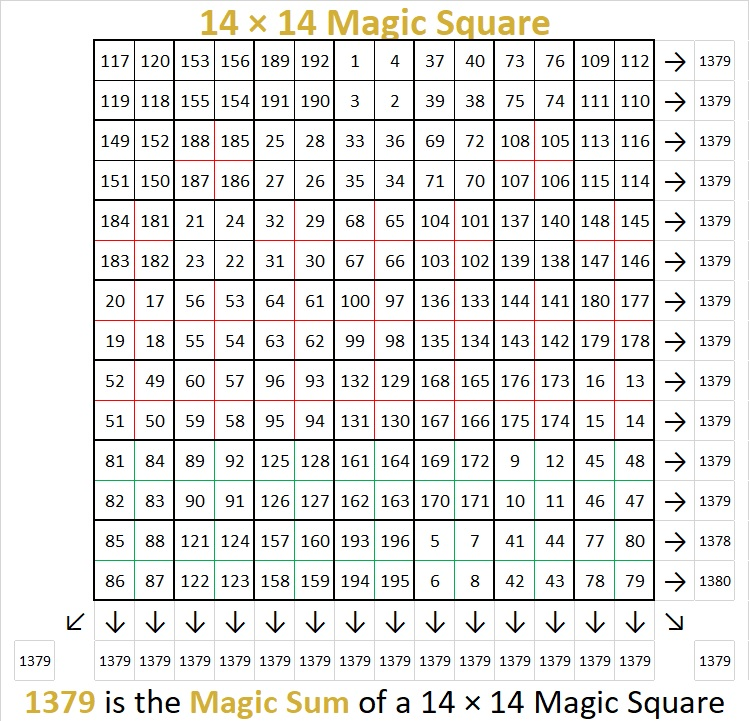# 1379 You Can Solve This Magic Square

I knew that 1379 was the magic sum of a 14 × 14 magic square because 14² = 196 and
(196)(197)/(2·14) = 1379.

I know how to solve a 4 × 4 magic square and when the dimensions of the magic square are odd numbers. I can also solve squares whose dimensions can be factored into any of those.  Clearly, the dimensions of a 14 × 14 magic square don’t qualify. I wondered if there was a simple way to solve it.I watched a video that explained how to use a 7 × 7 magic square to solve one that is
14 × 14
. You don’t have to watch the video to solve this magic square. Let me explain:

Notice that I’ve grouped the squares in the 14 × 14 magic square into 4 × 4 sub-grids. Now the magic square can behave more like a 7 × 7 magic square. Also, notice that the highest number in each sub-grid is 4 times the corresponding number in a 7 × 7 magic square.You should notice that many of the numbers appear in order along the diagonal of the 7  × 7 square. The trickiest part for me is always the upper right corner.

Unfortunately, the 4 × 4 sub-grids are not all the same. In the video, they were labeled X, Y, and Z. To make it simpler, I’ve color-coded them so that you can know how to place the four numbers in each sub-grid from lowest to highest.You can use this excel file,10 Factors 1373-1388 to first solve the 7 × 7 magic square and then use it and the three squares above to solve the 14 x 14 one. I would encourage you to give it a try! It is so satisfying to succeed!

Here’s a little more about the number 1379:

• 1379 is a composite number.
• Prime factorization: 1379 = 7 × 197
• 1379 has no exponents greater than 1 in its prime factorization, so √1379 cannot be simplified.
• The exponents in the prime factorization are 1, and 1. Adding one to each exponent and multiplying we get (1 + 1)(1 + 1) = 2 × 2 = 4. Therefore 1379 has exactly 4 factors.
• The factors of 1379 are outlined with their factor pair partners in the graphic below.1379 is the hypotenuse of a Pythagorean triple:
196-1365-1379 which is 7 times (28-195-197)